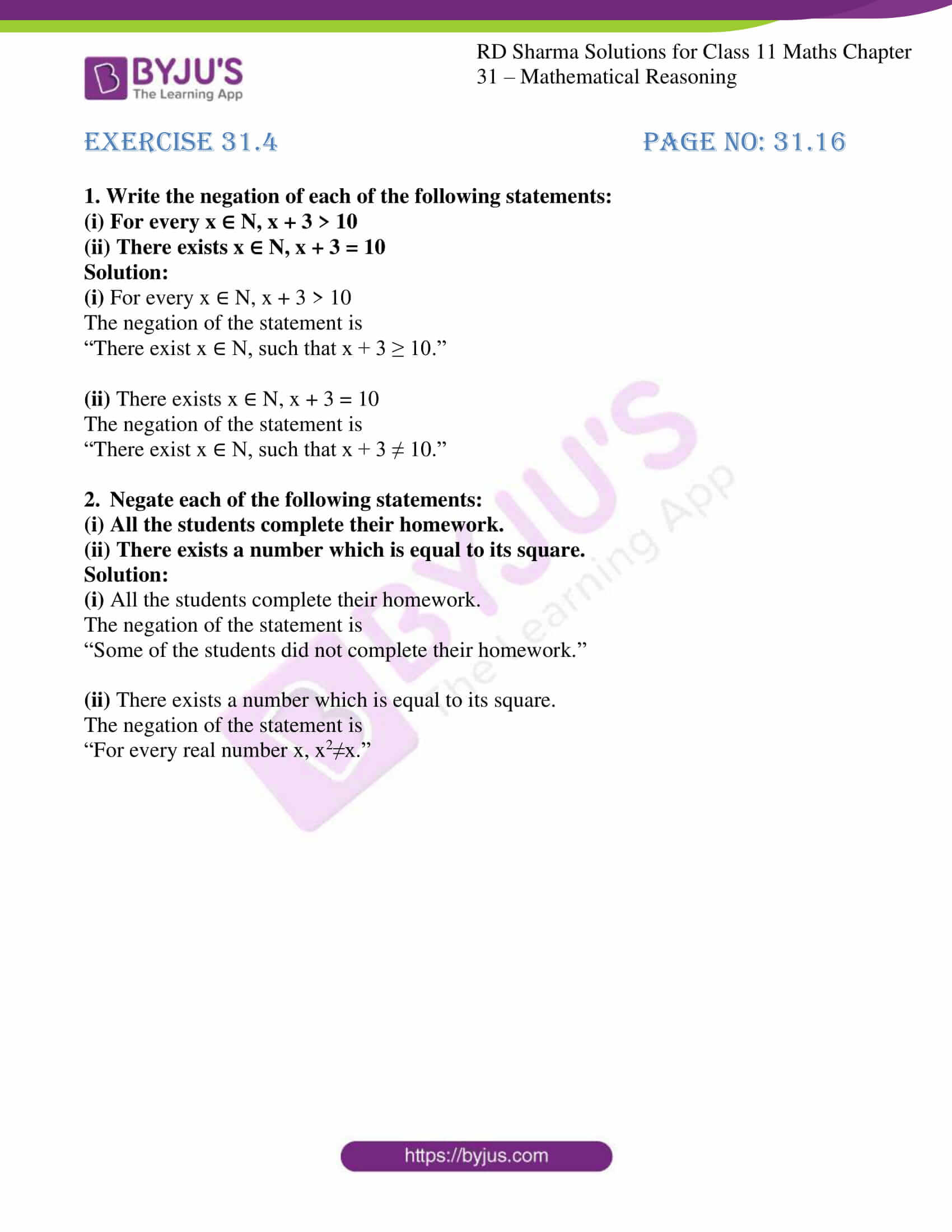# RD Sharma Solutions for Class 11 Chapter 31 - Mathematical Reasoning Exercise 31.4

In Mathematics, we come across many mathematical statements containing phrases “There exists” and “For every”. These two phrases are called quantifiers. In this exercise, we mainly deal with quantifiers. Experts have solved the exercise wise problems using simple techniques to help students improve their accuracy in solving problems. The solutions are free to download and the questions are systematically arranged for students to prepare at ease as well as to solve different types of questions. In order to score good marks, students are advised to prepare from RD Sharma Class 11 Maths Solutions which are readily available in the pdf format and can be downloaded from the links given below.

## Download the pdf of RD Sharma Solutions for Class 11 Maths Exercise 31.4 Chapter 31 – Mathematical Reasoning### Also, access other exercises of RD Sharma Solutions for Class 11 Maths Chapter 31 – Mathematical Reasoning

Exercise 31.1 Solutions

Exercise 31.2 Solutions

Exercise 31.3 Solutions

Exercise 31.5 Solutions

Exercise 31.6 Solutions

### Access answers to RD Sharma Solutions for Class 11 Maths Exercise 31.4 Chapter 31 – Mathematical Reasoning

#### EXERCISE 31.4 PAGE NO: 31.16

1. Write the negation of each of the following statements:
(i) For every x ∈ N, x + 3 > 10
(ii) There exists x ∈ N, x + 3 = 10

Solution:

(i) For every x ∈ N, x + 3 > 10

The negation of the statement is

“There exist x ∈ N, such that x + 3 ≥ 10.”

(ii) There exists x ∈ N, x + 3 = 10

The negation of the statement is

“There exist x ∈ N, such that x + 3 ≠ 10.”

2. Negate each of the following statements:
(i) All the students complete their homework.
(ii) There exists a number which is equal to its square.

Solution:

(i) All the students complete their homework.

The negation of the statement is

“Some of the students did not complete their homework.”

(ii) There exists a number which is equal to its square.

The negation of the statement is

“For every real number x, x2≠x.”